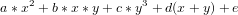# Could you please point me to an implementation of Formulation A in the factoring example?

Hi,

I'm trying to work on a problem which involves minimisation of polynomials which are generated on the fly. The closest example I could find was Formulation A in the Factoring examples.

Could someone point me to an implementation or point to docs which will help me feed polynomials to the DWave system?

Formulation A is provided in the Factoring Jupyter Notebook is an intuitive way of solving the factoring problem P = ab. In the case of factoring integers, this approach becomes very complex and resource-heavy, so a better approach involving AND gates (Formulation B) is presented in more detail.

If you're interested in trying out more general polynomial minimization problems, the general instructions provided in the Factoring Jupyter Notebook will work:

1. state equation as a minimization
2. represent integers as binary numbers
3. reduce to quadratic

For step (3), you can use the dimod function make_quadratic. If you are interested in seeing how this works, see the documentation on reducing polynomial order

After this step, you can use a D-Wave sampler to embed the BQM and run on the QPU (or a classical solver).

• I am not able to understand how a polynomial is supposed to fed to the make_quadratic function. From what I saw in the tests, {(0,): .5, tuple(): 1.3} is basically 0.5*x +1.3. Is this correct?

If the polynomial I am considering iswhat would the dictionary look like?

Thank you so much for your help.

There are a few key points to consider if you want to minimize a polynomial with a Binary Quadratic Model (BQM):

1. A BQM is Binary, so all your variables must evaluate to 1 or 0. The polynomial you provided above is alright as long as x and y are binary variables. Otherwise, you can expand integers to binary variables through the process described in Formulation A of the factoring notebook (e.g. x = x_0 + 2*x_1 + 4*x_2 + ...)
2. Since we are only trying to determine the values of your variables for which the polynomial is minimized, we can ignore the constant (variable "e" in your polynomial example above). This is necessary because BQMs do not have zero-order terms.
3. As mentioned in the factoring notebook, you can simplify the polynomial using the property of binary variables that x^2 = x.

Once your polynomial is in this format, you can use the dimod tool make_quadratic to reduce your polynomial to quadratic. The syntax you wrote above is correct except (following point #2 about zero-order terms) you can leave out the constant "1.3" term.

Following the steps above, the direct coding of your polynomial (assuming x and y are already binary variables) would be:

`poly = {(0,): (a+d), (1,): (c+d), (0,1): b}`

Note that this polynomial is already quadratic so there would be no need to pass it through make_quadratic.

• Thank you so much

• No problem Sadhana, I realize there is a lot of background information to take in. Please do share your progress, I'm sure your project will be interesting and helpful to other users as well.